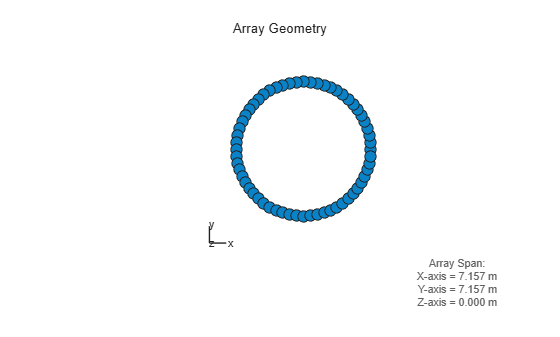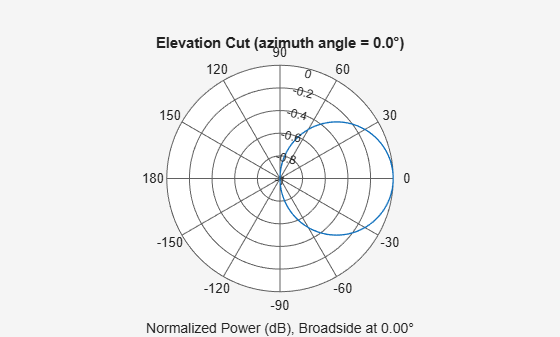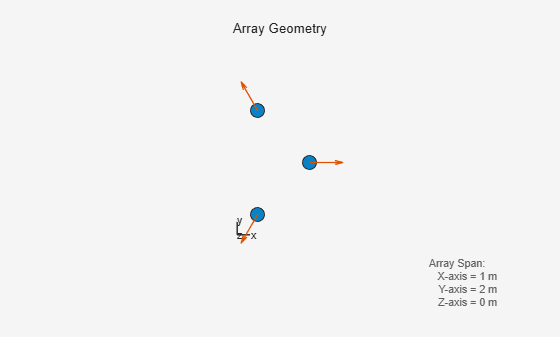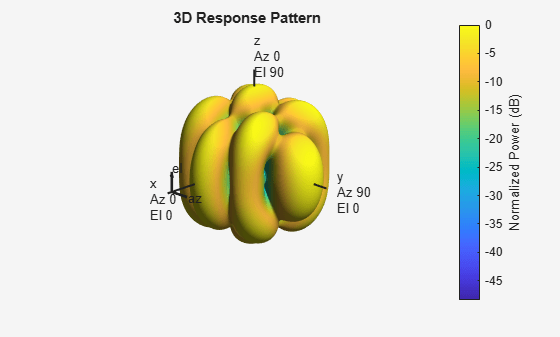Documentation

## Conformal Array

### Support for Arrays with Custom Geometry

The `phased.ConformalArray` object lets you model a phased array with arbitrary geometry. For example, you can use `phased.ConformalArray` to design:

• A planar array with a nonrectangular geometry, such as a circular array

• An array with nonuniform geometry, such as a linear array with variable spacing

• A nonplanar array

When you use `phased.ConformalArray`, you must specify these aspects of the array:

• Sensor element of the array

• Element positions

• Direction normal to each array element

### Create Default Conformal Array

To create a conformal array with default properties, use this command:

`array = phased.ConformalArray`
```array = phased.ConformalArray with properties: Element: [1x1 phased.IsotropicAntennaElement] ElementPosition: [3x1 double] ElementNormal: [2x1 double] Taper: 1 ```

This default conformal array consists of a single `phased.IsotropicAntennaElement` antenna located at the origin of the local coordinate system. The direction normal to the sensor element is 0° azimuth and 0° elevation.

### Uniform Circular Array Created from Conformal Array

This example shows how to construct a 60-element uniform circular array. In constructing a uniform circular array, you can use either the `phased.UCA` or the `phased.ConformalArray` System objects. The conformal array approach is more general because it allows you to point the array elements in arbitrary directions. A UCA restricts the array element directions to lie in the plane of the array. This example illustrates how you can use the `phased.ConformalArray `System object to create any other array shape. Assume an operating frequency of 400 MHz. Tune the array by specifying the arclength between the elements to be 0.5$\lambda$ where $\lambda$ is the wavelength corresponding to the operating frequency. Array elements lie in the x-y-plane. Element normal directions are set to $\left({\varphi }_{n},0\right)$ where ${\varphi }_{n}$ is the azimuth angle of the ${n}^{th}$ array element.

Set the number of elements and the operating frequency of the array.

```N = 60; fc = 400e6;```

Compute the element spacing in radians.

```theta = 360/N; thetarad = deg2rad(theta);```

Choose the radius so that the inter-element arclength is one-half wavelength.

```arclength = 0.5*(physconst('LightSpeed')/fc); radius = arclength/thetarad;```

Compute the element azimuth angles. Azimuth angles must lie in the range $\left(-18{0}^{\circ },18{0}^{\circ }\right)$.

```ang = (0:N-1)*theta; ang(ang >= 180.0) = ang(ang >= 180.0) - 360.0; array = phased.ConformalArray; array.ElementPosition = [radius*cosd(ang);... radius*sind(ang);... zeros(1,N)]; array.ElementNormal = [ang;zeros(1,N)];```

Show the UCA array geometry.

`viewArray(array)`Plot the array response pattern at 1 GHz.

```pattern(array,1e9,[-180:180],0,'PropagationSpeed',physconst('LightSpeed'),... 'CoordinateSystem','polar','Type','powerdb','Normalize',true)```### Custom Antenna Array

This example shows how to construct and visualize a custom-geometry array containing antenna elements with a custom radiation pattern. The radiation pattern of each element is constant over each azimuth angle and has a cosine pattern for the elevation angles.

Define the custom antenna element and plot its radiation pattern.

```az = -180:180; el = -90:90; fc = 3e8; elresp = cosd(el); antenna = phased.CustomAntennaElement('AzimuthAngles',az,... 'ElevationAngles',el,... 'MagnitudePattern',repmat(elresp',1,numel(az))); pattern(antenna,3e8,0,el,'CoordinateSystem','polar','Type','powerdb',... 'Normalize',true);```Define the locations and normal directions of the elements. All elements lie in the z-plane. The elements are located at (1;0;0) , (0;1;0), and (0;-1;0) meters. The element normal azimuth angles are 0°, 120°, and -120°, respectively. All normal elevation angles are 0°.

```xpos = [1 0 0]; ypos = [0 1 -1]; zpos = [0 0 0]; normal_az = [0 120 -120]; normal_el = [0 0 0];```

Define a conformal array with those elements.

```array = phased.ConformalArray('Element',antenna,... 'ElementPosition',[xpos; ypos; zpos],... 'ElementNormal',[normal_az; normal_el]);```

Plot the positions and normal directions of the elements.

```viewArray(array,'ShowNormals',true) view(0,90)``````pattern(array,fc,az,el,'CoordinateSystem','polar','Type','powerdb',... 'Normalize',true,'PropagationSpeed',physconst('LightSpeed'))```